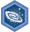## FANDOM

1,895 Pages

Algebra II: Systems of equations and inequalitiesDescription
Achieve mastery in all skills in Algebra II: Systems of equations and inequalities
Details
TypeEnergy point(s) awarded 0
Status Available
Disclosed?
Repeating?

This page contains exercises which pertain to the subcategory Algebra II: Systems of equations and inequalities. Mastering them awards user with the Challenge Patch Algebra II: Systems of equations and inequalities as well.

All items (15)

A
•Analyze the solutions of systems of equations graphically
C
•Checking solutions to two-variable linear equations
•Completing solutions of systems of inequalities
G
•Graphing systems of inequalities and checking solutions
I
•Intersecting functions
S
•Solving systems of equations graphically
•Solving systems of linear equations with elimination 1
•Solving systems of linear equations with elimination 2
•Solving systems of linear equations with substitution
•Solving systems of two linear equations
•Systems of equations word problems
•Systems of linear equations word problems
•Systems of nonlinear equations
•Systems with one, zero, or infinite solutions
T
•Two-variable linear inequalities word problems